Fingerings Home Octave 1: F  (C) Gb  (Db) G  (D) Ab  (Eb) A  (E) Bb  (F) B  (Gb) C  (G) Db  (Ab) D  (A) Eb  (Bb) E  (B) Octave 2+: F  (C) Gb  (Db) G  (D) Ab  (Eb) A  (E) Bb  (F) B  (Gb) C  (G) Db  (Ab) D  (A) Eb  (Bb) E  (B) F  (C) Gb  (Db) G  (D)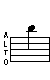B (Gb) - 2nd Octave## Trills

Trill - From Db
(See below for alternate)
Trill - From C
Db B (alt)   C B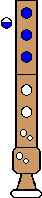Alternate Trill - From Db
Db BComments: advantage over normal trill - it ends on a normal B
Top

## Turned Trills

Turned Trill
Full step above, Full step below
Db B (alt) A B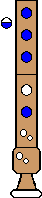Comments: End on the alternate B for 2 trills in a row.
Top

Turned Trill
Half step above; Full step below
C B A BTop

Turned Trill
Full step above; Half step below

(See below for alternate)
Db B (alt) Bb (alt) B (alt)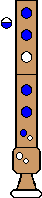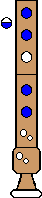Alternate
Turned Trill
Full step above; Half step below
Db B (alt) Bb (alt) B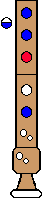Comments: Start on normal Db, go to normal B, then trill as shown. Good because it ends on normal B.
Top

Turned Trill
Half step above; Half step below
C B Bb (alt) BTop

## Regular Turns

Turn
Full step above; Full step below
Db B (alt) A BComments: You could also end on the alternate B
Top

Turn
Half step above; Full step below
C B A BTop

Turn
Full step above; Half step below
Db B Bb (alt) B